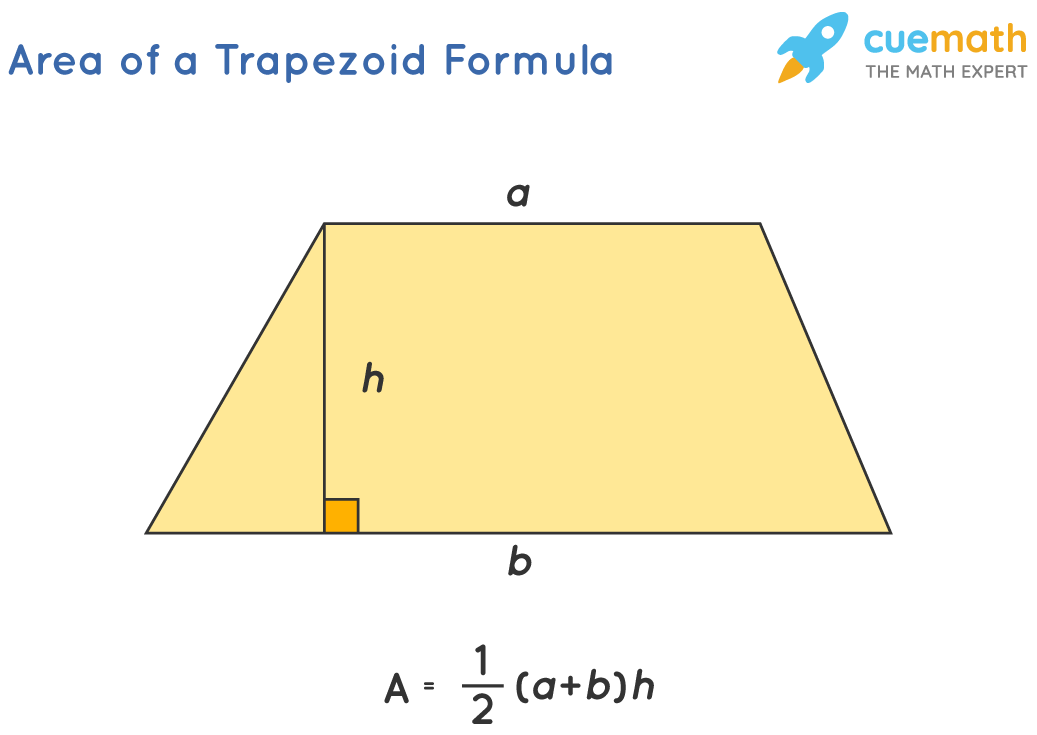# The Area of The Trapezoid

## Question: What is the area of the trapezoid?

trapezoid is a quadrilateral with one pair of parallel sides (which are known as bases).

## Answer: The area of a trapezoid is A = (1/2) × (a + b) × h

The area of the trapezoid is calculated by measuring the average of the parallel sides and multiplying it with its height.

## Explanation:

The area of a trapezoid can be shown in the figure given below:So, the area of a trapezoid is A = (1/2) × (a + b) × h

Here, a and b are the lengths of the two parallel sides, and 'h' is the height of the trapezoid (distance between two parallel sides).